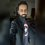# Help! Integration

If the value of

$$\displaystyle f(k) = \int_0^{\infty} \frac{x^{k}}{2x^{6} + 4x^{5} + 3x^{4} + 5x^{3} + 3x^{2} + 4x + 2} dx$$

Is minimum

Then find

$101k$, (k is an integer)Note by Krishna Sharma
6 years, 11 months ago

This discussion board is a place to discuss our Daily Challenges and the math and science related to those challenges. Explanations are more than just a solution — they should explain the steps and thinking strategies that you used to obtain the solution. Comments should further the discussion of math and science.

When posting on Brilliant:

• Use the emojis to react to an explanation, whether you're congratulating a job well done , or just really confused .
• Ask specific questions about the challenge or the steps in somebody's explanation. Well-posed questions can add a lot to the discussion, but posting "I don't understand!" doesn't help anyone.
• Try to contribute something new to the discussion, whether it is an extension, generalization or other idea related to the challenge.

MarkdownAppears as
*italics* or _italics_ italics
**bold** or __bold__ bold
- bulleted- list
• bulleted
• list
1. numbered2. list
1. numbered
2. list
Note: you must add a full line of space before and after lists for them to show up correctly
paragraph 1paragraph 2

paragraph 1

paragraph 2

[example link](https://brilliant.org)example link
> This is a quote
This is a quote
    # I indented these lines
# 4 spaces, and now they show
# up as a code block.

print "hello world"
# I indented these lines
# 4 spaces, and now they show
# up as a code block.

print "hello world"
MathAppears as
Remember to wrap math in $$ ... $$ or $ ... $ to ensure proper formatting.
2 \times 3 $2 \times 3$
2^{34} $2^{34}$
a_{i-1} $a_{i-1}$
\frac{2}{3} $\frac{2}{3}$
\sqrt{2} $\sqrt{2}$
\sum_{i=1}^3 $\sum_{i=1}^3$
\sin \theta $\sin \theta$
\boxed{123} $\boxed{123}$

Sort by:

I don't know whether I am right or not but I am getting $k=2$, here's a solution.

Differentiating both sides with respect to k we get :

$\large { f }^{ ' }(k)=\int _{ 0 }^{ \infty }{ \frac { ln(x){ .x }^{ k } }{ (2x^{ 6 }+4x^{ 5 }+3x^{ 4 }+5x^{ 3 }+3x^{ 2 }+4x+2) } dx }=I$

I differentiated here using newton leibnitz rule.

Splitting the integral into two parts we get :

$\large I={ f }^{ ' }(k)=\int _{ 0 }^{ 1 }{ \frac { ln(x){ .x }^{ k } }{ (2x^{ 6 }+4x^{ 5 }+3x^{ 4 }+5x^{ 3 }+3x^{ 2 }+4x+2) } dx } +\int _{ 1 }^{ \infty }{ \frac { ln(x){ .x }^{ k } }{ (2x^{ 6 }+4x^{ 5 }+3x^{ 4 }+5x^{ 3 }+3x^{ 2 }+4x+2) } dx }$

In the second part put $x=\frac{1}{y}$ to get :

$\large { f }^{ ' }(k)=\int _{ 0 }^{ 1 }{ \frac { ln(x){ .x }^{ k } }{ (2x^{ 6 }+4x^{ 5 }+3x^{ 4 }+5x^{ 3 }+3x^{ 2 }+4x+2) } dx } +\int _{ 0 }^{ 1 }{ \frac { -ln(y).y^{ 4-k } }{ (2y^{ 6 }+4y^{ 5 }+3y^{ 4 }+5y^{ 3 }+3y^{ 2 }+4y+2) } dy }$

Combining both integrals we get :

$\large { f }^{ ' }(k)=\int _{ 0 }^{ 1 }{ \frac { ln(x){ .(x }^{ k }-{ x }^{ 4-k }) }{ (2x^{ 6 }+4x^{ 5 }+3x^{ 4 }+5x^{ 3 }+3x^{ 2 }+4x+2) } dx }$

Equating the derivative equal to $0$ we get :

$k=4-k$

$\boxed{\Rightarrow k=2}$

I won't do the second derivative test as I know this has the minimum possible value.

- 6 years, 11 months ago

Is The answer is 202 means K=2 ??
( I have feeling That it might not be correct)

Since You Didn't Specify That 'K' is any real number or an integer So I have Assumed That
K is integer.. If it is real then please mention it.!!

- 6 years, 11 months ago

Question was integer type and we need to find K...I guess so it is integer. How do got 2?

- 6 years, 11 months ago

Well I first substitute $x=\frac { 1 }{ t }$. and then using Properties of integration I get :

$f(k)=\int _{ 0 }^{ \infty }{ \frac { { x }^{ k }+{ \frac { { x }^{ 4 } }{ { x }^{ k } } } }{ (2x^{ 6 }+4x^{ 5 }+3x^{ 4 }+5x^{ 3 }+3x^{ 2 }+4x+2) } dx }$.

Since Denominator is an $Palindrome$ Then it must be Products of Perfect squares. So Denominator is always Positive.

And To Minimize integral then We have To minimize The numerator. (For a Particular $x$)

So Apply

$AM\quad =\quad GM$. in numerator Keeping $x$ constant .

Or

Also thinks that in Numerator as 'k' increases First term is Increases and Second term is decreases
So Minimum Value of Numerator occurs when $first$ $term$=$Second$ $Term$. in Numerator

Or

You can also Prove it by using calculus That numerator is minimum when $K=2$. Hence The Integral also minimum.

This Gives $k=2$.

But I'am not sure that this method is correct or not.

- 6 years, 11 months ago

Very beautiful approach , really very nice

- 6 years, 8 months ago

@U Z thank you [ feeling blessed :) ]

- 6 years, 8 months ago

This was really great, hats off- 6 years, 8 months ago

Yes this is correct! My teacher also said to substitute $x = \frac {1}{y}$ You should have differentiated the function. $(f'(x) = 0$) would be more correct.